# Math Worksheets

#### Why Is Math So Important?

Math, a subject that not many students are fond of. The subject that involves numbers and lots of calculations is not something that people like. All those who are not 'fans' of this subject need to know that the concepts taught in it are frequently used in day-to-day life. Math is important, and you must realize its significance. Learning math is great for a child's mental development. As per studies, the children who do math have higher gray matter volume in the brain. It helps an individual tell you time. Most importantly, mathematics helps keep a check on your finances, something you will have to manage when you grow up. Did you know learning math could help you become a better cook or baker? It even enhances problem-solving skills in individuals. We have explored how to improve our math teaching and learning over these articles:

Top 10 Tips for Teaching Elementary Math
Teacher's Guide to Creating a Math Homework System
Application Strategies Help Math Students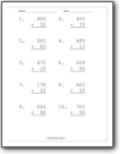Single, double, triple, and mixed digits on well spaced out sheets.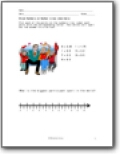###### Algebra

We focus on the common uses and start with single variables to advance to complex word problems.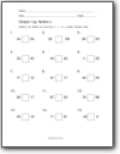###### Comparing Numbers

We practice working on determining values that are greater than, less than, or equal.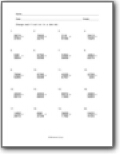###### Decimals

A nice mix of practice on converting values between decimals, fractions, and percentages. We also cover basic decimal value operations.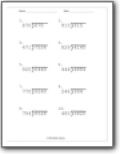###### Division

We start with a very simple operation of comparing division to sharing and grouping. We advance to complex operations.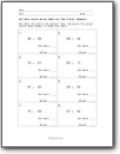###### Estimation

We show you how to use this skill to your advantage and process problems much quicker.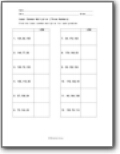###### Factoring

We show you how to determine the greatest common factor and least common multiplier between pairs of values.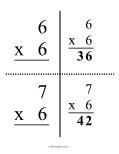###### Flashcards

A great technique to help improve your ability with all types of math operations.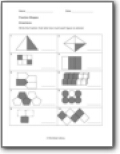###### Fractions

Learn how to convert between various values and perform all types of arithmetic.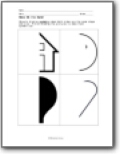###### Geometry

Students take time to work with angles, lines, and geometric shapes.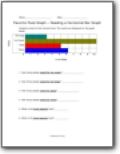###### Graphing

We start by learning to read all types of graphs and then we learn how to make our own.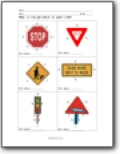###### Measurement

A focus on measures that sum up objects such as perimeter and area.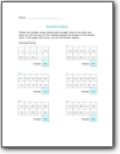###### Mixed Math

Basic arithmetic with all the operations and math combinations.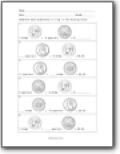###### Money Math

Learn how to work with currency.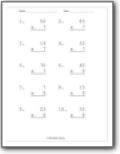###### Multiplication

Learn how to master this basic operation as we introduce it as repeated addition.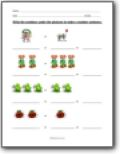###### Numbers

We work on number recognition and basic counting skills.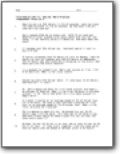###### Percentages

Learn to convert between percentage values and other number systems.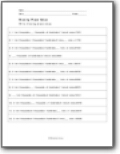###### Place Value

Learn to evaluate values by place and understand the significance of that numeral within the value.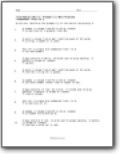###### Probability

Learn to accurately predict possible outcomes.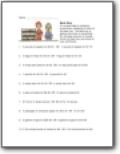###### Ratios

This set includes a large number of visuals to drive this concept home.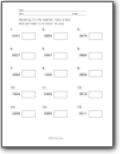###### Rounding

We work on rounding to the nearest whole value and thousandths through thousands places.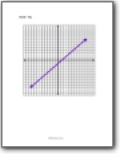###### Statistics

We learn how to manipulate and understand large data sets.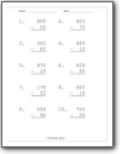###### Subtraction

We learn to perfect this simple operation even in complex situations.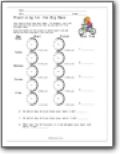###### Time

We learn how to tell time and apply it to complex word problems.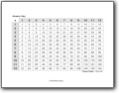###### Times Tables

A great method for helping you make multiplication automatic.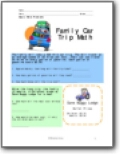###### Word Problems

We use problems that are related to real life situations. The problems span from very basic to complex.